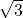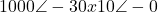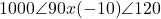# Open Delta Connection of Transformer: Calculation, Formula And Diagram (V-V Connection)

Contents

## What is an Open Delta Connection Transformer?

An open delta connection transformer uses two single-phase transformers to provide a three-phase supply to the load. An open delta connection system is also called a V-V system. Open delta connection systems are usually only used in emergency conditions, as their efficiency is low when compared to delta-delta (closed delta) systems (which are used during standard operations).

In subsequent discussion, we will explain this system with the help of some numerical values.

Suppose you have three single-phase transformers of 10 kVA each. They are connected in (both primary & secondary sides) in a delta connection, then it can be said they are connected in a closed delta system.

How much 3Ø balanced load can be supplied by this combination?

The answer is, this combination can supply 30 kVA, three-phase balanced load. Load on each transformer will be 10 kVA, i.e. each transformer is operating at its rated capacity.

Now, let one transformer is damaged and disconnected due to repairing purpose. Now the remaining system will work as an open delta system (i.e. in the open delta, we have two single-phase transformers).

Now, how much 3Ø balanced load can be supplied by this combination?

Answer is, now we have two single-phase transformers of 10 kVA, but we are not able to supply 20 kVA, 3Ø balanced load.

This combination can supply a maximum of 17.32 kVA three-phase balanced load. Load on each transformer will be 10 kVA, i.e. each transformer is operating at its rated capacity.

The efficiency of this open delta system will be less as compared to the closed delta system. This is because both transformers are operating at rated capacity (i.e. 10 kVA), so their losses will be full load losses, but the output is reduced (output is 17.32 kVA instead of 20 KVA).

If the output of open delta system is possible to be 20 kVA, then the efficiency of closed delta & open delta systems will be same, and in the whole world, to supply three phase load, instead of three single phase transformers, two single phase transformers may be sufficient.

So, you are able to continue three phase supply as open delta system but at reduced efficiency.

So how can the value of 17.32 kVA be calculated? We will breakdown this calculation in the next section.

## Open Delta Transformer Calculations (Example Problem)

Capacity of open delta system =x rating of one transformer =x 10 kVA= 17.32 kVA.

OR

Capacity of open delta system = 0.577 x rating of closed delta system=0.577 x 30 kVA= 17.32 kVA.

(The total load carried by open delta system is 57.7% of closed delta system)

You can better understand the above calculation with the circuit diagram given in the figure below:

The above figure shows the connection diagram of an open delta system. In this diagram, a three-phase unity PF load (resistive load) is supplied by two transformers. Each transformer is rated as 10 kVA (current rating is 10 A and voltage rating is 1000 V). It can be observed that at point ‘A’ Kirchoff’s Current Law (KCL) is satisfied. Also, recall that phase difference between Line current and Line-Line voltage (phase to phase voltage) is 30°. It can be observed, three-phase load is consuming power (in kVA) =x1000x10=17320 VA=17.32 kVA

Load on each transformer is 10 kVA (Voltage across each transformer is 1000 V, current flowing through each transformer is 10 A). Therefore each transformer is operating at 10 kVA, but load supplied is 17.32 kVA (less than 20 kVA).

If you will calculate load in kW (active power) then you will find that Load on both the transformers will be equal to three phase load (in kW). From the Figure-1, it can be calculated that load supplied by transformer-1 is: VI*==8660.2-j5000

VI*==8660.2+j5000

(In the calculation of transformer-2, Sign of current is minus (it is -10 instead of 10), readers can understand it themselves)

So, it can be seen that load on both the transformers is 8660.2 Watt (8.66 kW) and they are supplying a 3Ø load of 17.32 kW.

One transformer is generating reactive power 5000 VAR (5 kVAR), another is consuming the same amount of reactive power. Reactive power consumed by the load is zero (as it is resistive load, power factor is unity). Since there is an exchange of reactive power between both the transformers, due to this reason total kVA rating of transformers is more than kVA rating of load.

Suppose the load is not resistive, its power factor is cosø, then power factor of both the transformers can be calculated to be cos(30+ø) and cos(30-ø). On that basis power supplied by transformers can be calculated.

In the present example, load is resistive, power factor is unity, hence ø=0, therefore active power (kW power) supplied by transformer is 10 kVAxcos30=8.66 kW.

Want To Learn Faster? 🎓
Get electrical articles delivered to your inbox every week.
No credit card required—it’s 100% free.